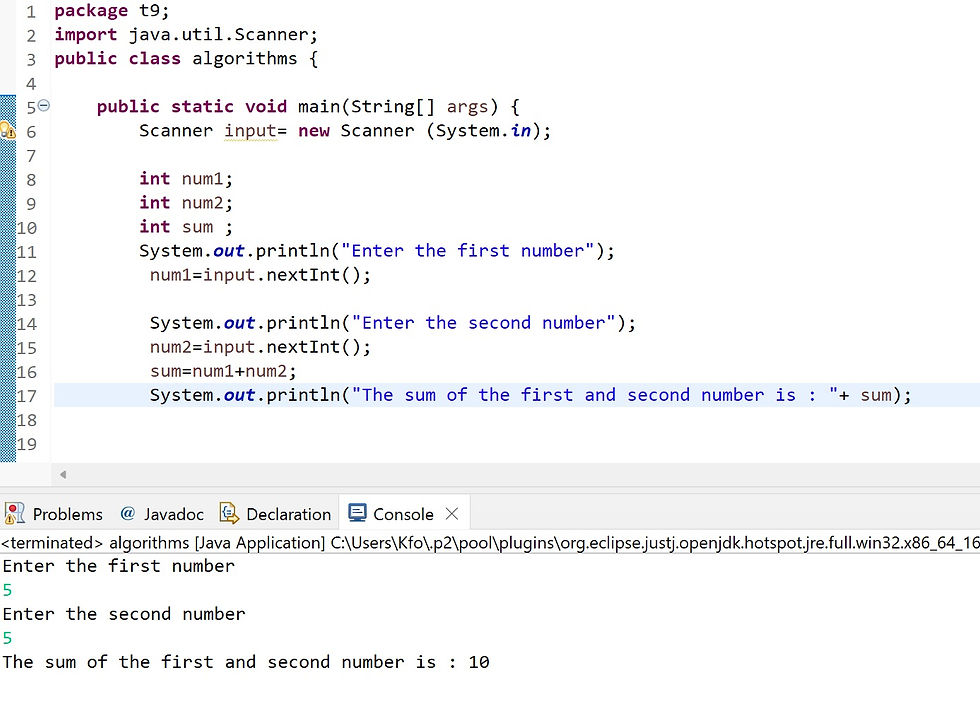•A.razaq

# T9-Java algorithms

java algorithm is a procedure for solving a problem in terms of the actions to execute and the order in which these actions execute.

algorithm example:

1. Start

2. Declare variables num1, num2 and sum.

3. Read values num1 and num2.

4. Add num1 and num2 and assign the result to sum. (sum←num1+num2)

5. Display sum.

6. Stop.

now lets make this algorithm into code

first we add the scanner class as we learned previously

and these are the precise steps of our algorithm

``` int num1;
int num2;
int sum ;

num1=input.nextInt();
num2=input.nextInt();
sum=num1+num2;
System.out.println(sum); ```now in order to make our program more user friendly ( not part of the algorithm) we add extra lines

to ask the user to input the values of num1 and num2

so the final code will be like this

``` int num1;
int num2;
int sum ;
System.out.println("Enter the first number");
num1=input.nextInt();

System.out.println("Enter the second number");
num2=input.nextInt();
sum=num1+num2;
System.out.println("The sum of the first and second number is : "+ sum);```-------------

Pseudocode is an informal language that helps you develop algorithms without having to worry about the strict details of Java language syntax, Pseudocode does not execute on computers.

Example:

• If student's grade is greater than or equal to 60

• Print "passed"

• else

• Print "failed"

we will learn all about this in the next tutorial